Showing 131 to 140 of 346 results.
0%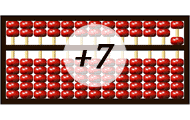Learn to count addition on an abacus. In order to be able to perform addition on an abacus you need to master the abacus basics. In this game you practice to carry extra digits when adding numbers.
0%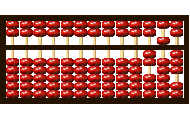## Abacus - Basics

Learn to count on an abacus online. In order to be able to count on an abacus you have to master the basics. In this game you train the values of the beads, the importance of the beam and the rods.
Logical thinking Mental calculation Abacus
0%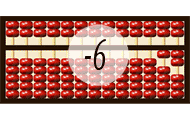## Abacus - Subtraction

Practice subtraction on the abacus. In this game you practice to borrow when performing math calculation with subtraction. Please play the other abacus games before playing this game for better unders
Logical thinking Subtraction Abacus
0%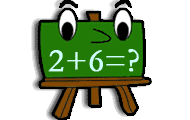Practice addition in math game with several levels of increasing difficulty. Add two numbers and find the correct sum.
35 game levels. Kindergarten - Sixth grade
0%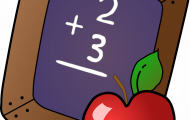This game will help students build fluency when adding within 10.
6 game levels. Kindergarten - First grade
0%## Balance the Equations

Use your addition and subtraction skills to balance the equations and win the game!
0%## Convert fractions, percentages and decimals

Practice convert a number between fraction, percentage and decimal. Includes the ten steps from a whole of one-tenth.
Fractions Percents Decimals Conversion
0%## Count money

Look at the notes and coins and add up the sum. Practicing basic knowledge in how to count money with increasing difficulty. This knowledge game uses all valid denominations of the Swedish krona, U.S.
Money Counting Mental calculation
0%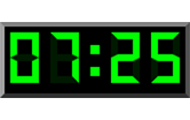## Digital clock in English

Practice the digital clock with 24 hours. Read a written time and find the corresponding time among the three digital clocks.
20 game levels. Kindergarten - Sixth grade
Clock Digital clock Numbers
0%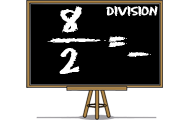## Division

Practice division, ie dividing two numbers. Find the quotients of the numerators divided by the denominators in this math game.
Numbers Division Mental calculation
Most played
1.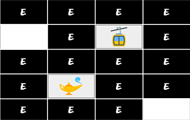Brain training
2.Brain training
3.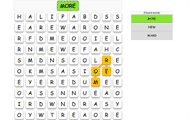English
4.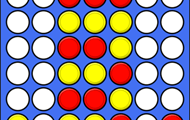Brain training
5.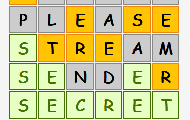English